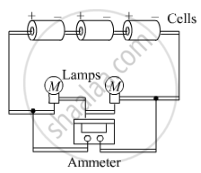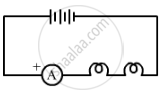Share

# A Student Made an Electric Circuit Shown Here to Measure the Current Through Two Lamps. Are the Lamps in Series Or Parallel? (A) Are the Lamps in Series Or Parallel? (B) the Student Has Made a Mistake in this Circuit. What is the Mistake? (C) Draw a Circuit Diagram to Show the Correct Way to Connect the Circuit. Use the Proper Circuit Symbols in Your Diagram. - CBSE Class 10 - Science

#### Question

A student made an electric circuit shown here to measure the current through two lamps.

Are the lamps in series or parallel?

(a) Are the lamps in series or parallel?
(b) The student has made a mistake in this circuit. What is the mistake?
(c) Draw a circuit diagram to show the correct way to connect the circuit. Use the proper circuit symbols in your diagram.#### Solution

(a) The lamps are connected in series.
(b) The student has connected the ammeter in parallel with the lamps. It should be connected in series.
(c) The correct diagram that shows how to connect the circuit:Is there an error in this question or solution?

#### Video TutorialsVIEW ALL 

Solution A Student Made an Electric Circuit Shown Here to Measure the Current Through Two Lamps. Are the Lamps in Series Or Parallel? (A) Are the Lamps in Series Or Parallel? (B) the Student Has Made a Mistake in this Circuit. What is the Mistake? (C) Draw a Circuit Diagram to Show the Correct Way to Connect the Circuit. Use the Proper Circuit Symbols in Your Diagram. Concept: Electric Current and Circuit.
S